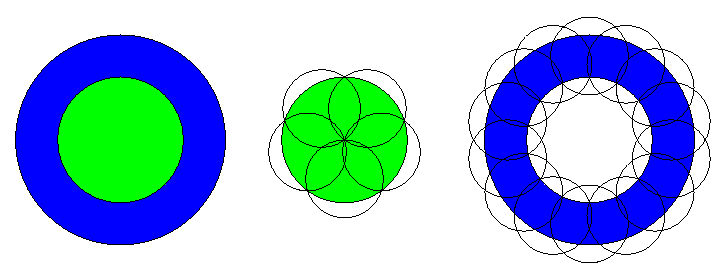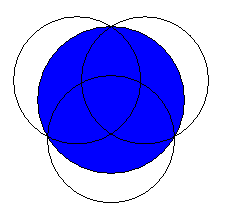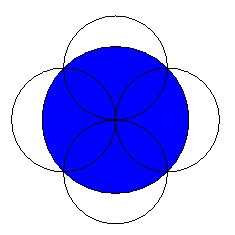### Problem Statement

We are given a disc of radius discRadius and want to cover it using numDiscs small discs - i.e., place the small discs flat above the original disc in such a way that no point of the original disc is visible (assuming the small discs are opaque). All smaller discs we use for the covering have the same radius.

We perform the covering using the following procedure:

1. In the first step, we split the disc into several rings using concentric circles (with the same center as the original disc). Note that the innermost "ring" will have an inner radius 0, and thus will actually be a disc. We are free to split the disc into as many rings and at whatever positions we want (i.e., use circles of arbitrary radii). The example below shows a disc split into three rings (blue, green and red).
2. In the second step, we regard each of the rings independently, and try to cover each of them using some number of the small discs. We can either use one small disc, which has to be centered at the center of the original disc, or use three or more discs, whose centers must be the vertices of a regular polygon centered at the center of the original disc. The example below shows one such case: The innermost ring (red, actually a disc) is covered by one small disc (which has the same radius as the red disc), the green ring is covered by 6 small discs centered at the vertices of a regular hexagon around the center, and the outermost blue ring is covered by 12 small discs centered at the vertices of a regular 12-gon. Please note that we try to cover each ring totally independently of the others, i.e., ignore that parts in one ring may have been covered already by the discs used to cover another ring (in the example below, the discs used to cover the green ring would also cover parts of the blue and red rings, but we ignore this fact).In summary, we have the freedom to choose the number and size of the rings that the original disc is split into, how many of the numDiscs discs are used for covering each of the rings, and the distance from the center at which the small discs for each ring are placed. We will have to choose these parameters such that a covering using this procedure is possible using the smallest possible discs.

Given discRadius, the radius of the original disc, and numDiscs, the total number of small discs to use, return the minimum radius that the small discs must have so that a covering using the above procedure is possible.

The example shown above is only one possibility to cover the original disc using 19 small discs (which turns out to be the optimal one for the used procedure). The figure below shows as an example another (non-optimal) possibility: here, the original disc is split only into two rings, and 5 of the small discs are used to cover the inner (green) ring, the other 14 small discs are used to cover the outer (blue) ring. It is clear here that too much space is wasted in the outer ring (that ring was chosen too narrow), so this can not be an optimal solution.### Definition

 Class: DiscCover Method: minRadius Parameters: double, int Returns: double Method signature: double minRadius(double discRadius, int numDiscs) (be sure your method is public)

### Notes

-When covering one ring with small discs, we ignore that there are parts of this ring covered already by the discs used for other rings.
-The innermost "ring" will be a disc (i.e. the inner radius will be 0).
-There may be just one "ring" in total (i.e. all smaller discs build a single regular polygon around the center).
-Your return value must have an absolute or relative error less than 1e-9.

### Constraints

-discRadius is between 1.0 and 1000.0, inclusive.
-numDiscs is between 1 and 2000, inclusive.

### Examples

0)

 `1000.0` `1`
`Returns: 1000.0`
 Here we can use only one disc to cover the given one. This must be at least as large as the original one.
1)

 `500.0` `2`
`Returns: 500.0`
 Using a second disc doesn't help much.
2)

 `100.0` `3`
`Returns: 86.60254037844385`
 Three discs of radius r placed in a triangle configuration around the center can cover a disc of maximum radius 2 * r / sqrt(3) (the centers of the small discs must be placed in a distance of 2 * r / 3 from the centers to achieve this maximum). So, to cover a disc of radius discRadius, we need three discs of radius discRadius * sqrt(3) / 2. This optimum is shown in the figure below:3)

 `100.0` `4`
`Returns: 70.71067811865474`
 This is the traditional game that can be found in some amusement parks, where you have to cover a disc using four smaller ones. The small discs must be at least 0.707 ( = sqrt(2)/2 ) times smaller than the disc to cover. The optimal configuration is shown below:4)

 `200.0` `19`
`Returns: 59.085199376486976`
 The example from the problem statement. The first configuration given in the problem statement is the optimal one for 19 discs.

#### Problem url:

http://www.topcoder.com/stat?c=problem_statement&pm=4452

#### Problem stats url:

http://www.topcoder.com/tc?module=ProblemDetail&rd=8093&pm=4452

gepa

#### Testers:

PabloGilberto , lbackstrom , Yarin , Olexiy

#### Problem categories:

Dynamic Programming, Geometry, Math